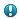English (English) - Change language

# Hypotheses for all types of elements

A hypothesis for an element can be either found or not found. A found hypothesis, as a rule, is formulated on the basis of one or several image objects or their fragments (an exception is the White Gap element which may contain no image objects at all).

A found hypothesis for a simple element is always a continuous region. Hypotheses for White Gap, Separator, and Barcode elements are always single rectangles.

If a hypothesis is formulated on the basis of a set of detected image objects, e.g. a hypothesis for a Paragraph element, the region of the hypothesis will be built on the basis of the array of rectangles of the detected objects. The region created in this way can be obtained by the Region method of the hypothesis. When displaying the region o f the hypothesis on the image, or when creating a block on the basis of the hypothesis, the boundaries of the region do not exactly follow the boundaries of each rectangle. Instead, the boundaries of the search area are smoothed out for better visualization. A region created in this way can be obtained using the SimplifiedRegion method of the hypothesis. The Region and SimplifiedRegion methods can be used either in the Advanced pre-search relations field on the Advanced tab (when describing the search area of the element), or in the Region expression field when describing the properties of the block and using the already found elements.

If a hypothesis was not found, it coincides with the fuzzy rectangle of its search area. When displaying a non-found hypothesis on the image, the program will draw the external rectangle of the fuzzy rectangle.

The Left, top, Right, and Bottom boundaries of any hypothesis are ranges. For each found hypothesis, the range degenerates to a point, i.e. Left.Start = Left.End, top.Start = top.End, etc. For a non-found hypothesis, the ranges of the boundaries coincide with the ranges of its search area.

Accessing generated hypotheses and their properties in the code of the corresponding dialog boxes of blocks and elements provides additional tools for setting up the FlexiLayout. Element hypotheses can be accessed by the names of the elements. To access a hypothesis for the current element in the Advanced post-search relations field, you can use only the name of the property.

Hypotheses and their properties are accessed in read-only mode. Hypotheses for elements can be accessed in the Advanced pre-search relations and Advanced post-search relations fields on the Advanced tab of the element's Properties dialog box as well as in the Region expression field in the block's Properties dialog box. In Advanced pre-search relations, you can access the generated hypotheses (and their properties) for all the elements located above the current element in the FlexiLayout tree. In Advanced post-search relations, you can access the generated hypotheses for the elements located above the current element in the FlexiLayout tree and the hypothesis for the current element itself. In Region expression, you can access the hypotheses (and their properties) for all the elements.

The table below lists the properties of hypotheses for all types of element.

 Property Description Logic IsNull() Whether this is a null hypothesis (if the element has been detected, IsNull property of the hypothesis is True). Logic IsFound() Whether the hypothesis is not null (if the element has not been detected, IsNull property of the hypothesis is False). Equivalent to IsNull. XInterval Left() The left boundary of the hypothesis, horizontal range in global coordinates. YInterval top() The top boundary of the hypothesis, vertical range in global coordinates. XInterval Right() The right boundary of the hypothesis, horizontal range in global coordinates. YInterval Bottom() The bottom boundary of the hypothesis, vertical range in global coordinates. XInterval XCenter() The X-coordinate of the center of the hypotheses, horizontal range in global coordinates. YInterval YCenter() The Y-coordinate of the center of the hypotheses, vertical range in global coordinates. DistInterval Width() The width of the hypothesis in global coordinates, range of distances. DistInterval Height() The height of the hypothesis in global coordinates, range of distances. FuzzyRect FuzzyRect() Fuzzy rectangle of the hypothesis in global coordinates. Rect Rect() The rectangle circumscribing the hypothesis in global coordinates. Coincides with the external rectangle of the fuzzy rectangle. Region Region() The region of the hypothesis in global coordinates. The method is not supported for Group elements. Region SimplifiedRegion() The region of the hypothesis in global coordinates simplified for better visual representation. The method is not supported for Group elements. ImageObjectSet Set() The image objects which are part of the hypothesis. ImageObjectSet ExcludeSet() The image objects which are in the region excluded from the search area by methods of type Exclude. RectArray Rects() The array of the rectangles included in the hypothesis. For most elements, it coincides with the array of the rectangles enclosing detected objects. For White Gap and Region elements, no objects are available. Int HypothesesCount() The number of hypothesis which can be formulated for the element in the search area.Important!The function may only be used if the maximum and minimum numbers of pages in the document are set to 1, i.e. the detected document has only one page. If you use this function for a multi-page document, matching a FlexiLayout will return an error. PageInterval Pages() The range of pages where the hypothesis is to be found. PageArea PageAreaGlobal( Int PageNumber ) Returns the part of the hypothesis located on the given page in global coordinates. PageArea PageAreaLocal( Int PageNumber ) Returns the part of the hypothesis located on the given page in the local coordinates of the page.

### Methods Region Region() and Region SimplifiedRegion()compared

14.01.2021 14:17:19